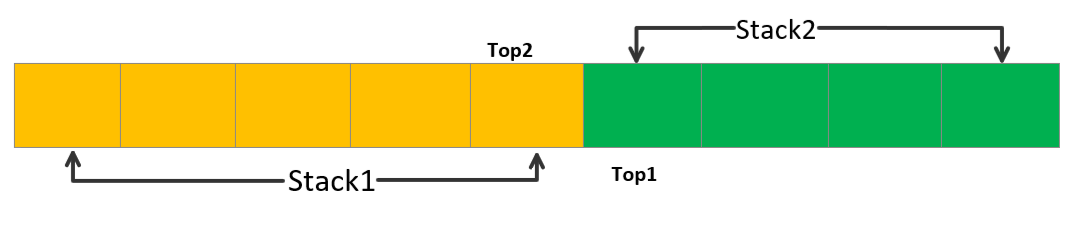# Two stacks in an array

Problem Statement: You need to try implementing 2 stacks in a single array.

Example:

```push1(10): Insert 10 in stack1
push2(21): Insert 21 in stack2
pop2(): Pop top element from stack2
```

Approaches

To solve this problem, we can use 2 approaches. One is space efficient and one is not.

Approach 1: Divide the array into two equal halves

In this approach, we’ll divide the array into two equal halves.

One half would be assigned to stack1 and one half would be assigned to stack 2.

The following diagram explains the above approachLet’s try coding this approach. Here we’ll use class twoStack to build our two-stack array.

Code:

## C++ Code

``````#include <iostream>
using namespace std;

class twoStack {
int * arr;
int size;
int top1;
int top2;
public:
twoStack(int n) {
arr = new int[n];
size = n;
top1 = n / 2 + 1;
top2 = n / 2;
}
void pushStack1(int data) {
if (top1 > 0) {
top1--;
arr[top1] = data;
cout << "Element pushed" << "\n";
} else {
cout << "Stack Overflow" << "\n";
}
}
void pushStack2(int data) {
if (top2 < size - 1) {
top2++;
arr[top2] = data;
cout << "Element pushed" << "\n";
} else {
cout << "Stack Overflow" << "\n";
}
}
void popStack1() {
if (top1 <= size / 2) {
top1++;
cout << "top element popped" << "\n";
} else {
cout << "Stack Underflow" << "\n";
}
}
void popStack2() {
if (top2 >= size / 2 + 1) {
top2--;
cout << "top element popped" << "\n";
} else {
cout << "Stack underflow" << "\n";
}
}
};
int main() {
twoStack ts(5);
ts.pushStack1(10);
ts.pushStack1(22);
ts.popStack1();
ts.popStack2();
ts.popStack1();
}
``````

Output:

Element pushed
Element pushed
top element popped
Stack underflow
top element popped

## Java Code

``````import java.util.*;

class TUF{
public static void main(String args[]) {
twoStack ts=new twoStack(5);
ts.pushStack1(10);
ts.pushStack1(22);
ts.popStack1();
ts.popStack2();
ts.popStack1();
}
}
class twoStack {
static int arr[];
static int size;
static int top1;
static int top2;

twoStack(int n) {
arr = new int[n];
size = n;
top1 = n / 2 + 1;
top2 = n / 2;
}
static void pushStack1(int data) {
if (top1 > 0) {
top1--;
arr[top1] = data;
System.out.println("Element pushed");
} else {
System.out.println("Stack Overflow");
}
}
static void pushStack2(int data) {
if (top2 < size - 1) {
top2++;
arr[top2] = data;
System.out.println("Element pushed");
} else {
System.out.println("Stack Overflow");
}
}
static void popStack1() {
if (top1 <= size / 2) {
top1++;
System.out.println("top element popped");
} else {
System.out.println("Stack Underflow");
}
}
static void popStack2() {
if (top2 >= size / 2 + 1) {
top2--;
System.out.println("top element popped");
} else {
System.out.println("Stack underflow");
}
}
}
``````

Output:

Element pushed
Element pushed
top element popped
Stack underflow
top element popped

Note: In this approach, there is a problem. Stackoverflow errors would be really common. Let’s understand this with an example.

Suppose you insert elements in stack1 and insert no elements in stack2. If we try inserting more elements in stack1, then the StackOverflow error would be raised since stack1 is full, but if we notice there is still space left in the array.

Now let’s discuss another efficient approach.

### Approach 2: Efficient Approach

This approach is a dynamic approach, in terms of stack but static in terms of the array.

Here if the number of elements is greater than the half of the array in one stack, we would still insert more elements until we reach the boundary of the other stack top pointer.

Here, we assign the top pointer of stack1 to -1 and the top pointer of stack2 to the array’s size.

The following code explains the above approach

Code:

## C++ Code

``````#include <iostream>
using namespace std;

class twoStack {
int * arr;
int size;
int top1;
int top2;
public:
twoStack(int n) {
arr = new int[n];
size = n;
top1 = -1;
top2 = size;
}
void pushStack1(int data) {
if (top1 < top2 - 1) {
top1++;
arr[top1] = data;
cout << "Element pushed" << "\n";
} else cout << "Stack Overflow" << "\n";
}
void pushStack2(int data) {
if (top1 < top2 - 1) {
top2--;
arr[top2] = data;
cout << "Element pushed" << "\n";
} else cout << "Stack Overflow" << "\n";
}
void popStack1() {
if (top1 >= 0) {
top1--;
cout << "Element popped" << "\n";
} else cout << "Stack Underflow" << "\n";
}
void popStack2() {
if (top2 < size) {
top2++;
cout << "Element Popped" << "\n";
} else cout << "Stack Underflow" << "\n";
}
};
int main() {
twoStack ts(6);
ts.pushStack1(10);
ts.popStack2();
return 0;
}
``````

Output:

Element pushed
Stack Underflow

## Java Code

``````
import java.util.*;

class TUF{
public static void main(String args[]) {
twoStack ts= new twoStack(6);
ts.pushStack1(10);
ts.popStack2();

}
}
class twoStack {
static int arr[];
static int size;
static int top1;
static int top2;

twoStack(int n) {
arr = new int[n];
size = n;
top1 = -1;
top2 = size;
}
static void pushStack1(int data) {
if (top1 < top2 - 1) {
top1++;
arr[top1] = data;
System.out.println("Element pushed");
} else System.out.println("Stack Overflow");
}
static void pushStack2(int data) {
if (top1 < top2 - 1) {
top2--;
arr[top2] = data;
System.out.println("Element pushed");
} else System.out.println("Stack Overflow");
}
static void popStack1() {
if (top1 >= 0) {
top1--;
System.out.println("Element popped");
} else System.out.println("Stack Underflow");
}
static void popStack2() {
if (top2 < size) {
top2++;
System.out.println("Element Popped");
} else System.out.println("Stack Underflow");
}
}
``````

output:

Element pushed
Stack Underflow

This approach is super efficient when you want to dynamically expand the stack in which an element needs to be inserted when the top pointer exceeds half of the array.